# Print all K-sum Paths in The Binary Tree

In this article, we are going to see how to find all the path in a given binary tree which has path sum as K?
Submitted by Radib Kar, on August 29, 2020

Here, we are going to see how we can find all paths having sum K in the given binary tree. Here, paths need not have to from root to leaf but need to be downwards.

In the below example,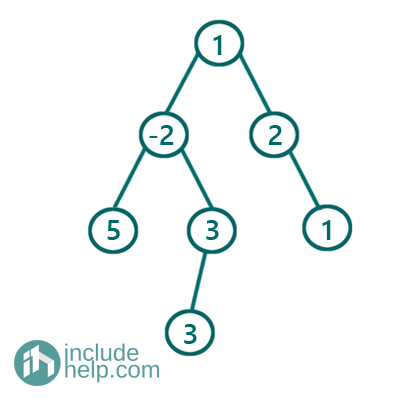Now, if K is 4, then we have the following paths:

[1, -2, 5]
[-2, 3, 3]
[1, 2, 1]

Solution:

Here, since the paths don't necessarily to be from root to leaf path, actually we need to generate all path and need to check whether the path sum is equal to k or not. That's why we will use backtracking.

The algorithm is like below:

We need a recursive function to store all paths and which will backtrack

Prerequisite: a vector named paths to store the path, a recursive function print_k_Sum_Path_Recur  that will store paths and will check the sum of the nodes on the path

```Function print_k_Sum_Path_Recur(root, paths, k){
1)  If the root is NULL
return;
2)  Add the current node to the path list
3)  recur for the left subtree
4)  Recur for the right subtree
5)  Find all paths stored in the current vector
paths having sum k & print those
6)  To backtrack remove the current node
```

C++ Implementation:

```#include <bits/stdc++.h>
using namespace std;

// tree node is defined
class TreeNode {
public:
int val;
TreeNode* left;
TreeNode* right;
TreeNode(int data)
{
val = data;
left = NULL;
right = NULL;
}
};

void printKpath(vector<int> path, int start)
{
for (int i = start; i < path.size(); i++) {
if (i == path.size() - 1)
cout << path[i] << endl;
else
cout << path[i] << "->";
}
}

void print_k_Sum_Path_Recur(TreeNode* root, vector<int>& paths, int k)
{
if (!root)
return;
//add current node to the path list
paths.push_back(root->val);

//recur for the left subtree
print_k_Sum_Path_Recur(root->left, paths, k);
//recur for right subtree
print_k_Sum_Path_Recur(root->right, paths, k);

//print all the paths from the path list having sum k
int sum = 0;
for (int i = paths.size() - 1; i >= 0; i--) {
sum += paths[i];
if (sum == k) {
printKpath(paths, i);
}
}
//backtrack
paths.pop_back();
}
//function to find all k sum paths
void print_k_Sum_Path(TreeNode* root, int k)
{
vector<int> path;
print_k_Sum_Path_Recur(root, path, k);
}

int main()
{
//building the tree like the example
TreeNode* root = new TreeNode(1);

root->left = new TreeNode(-2);
root->right = new TreeNode(2);
root->left->left = new TreeNode(5);
root->left->right = new TreeNode(3);
root->left->right->left = new TreeNode(3);
root->right->right = new TreeNode(1);

int k = 4;

print_k_Sum_Path(root, k);

return 0;
}
```

Output:

```1->-2->5
-2->3->3
1->2->1
```

Dry run:

Below we have shown glimpses of dry run to show how it works

Initially, the vector, paths, is empty and we call print_k_Sum_Path_Recur(root, paths, k)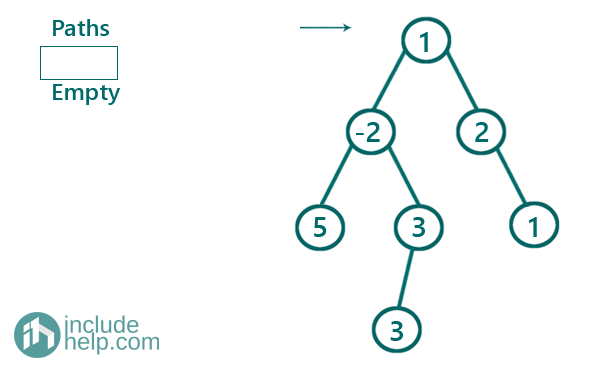Now,

The root is not NULL, so we add root to the vector paths and recur for the left subtree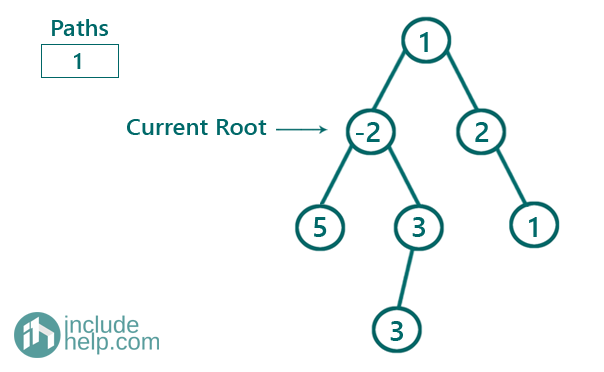Again,

The root is not NULL, so we add root to the vector paths and recur for the left subtree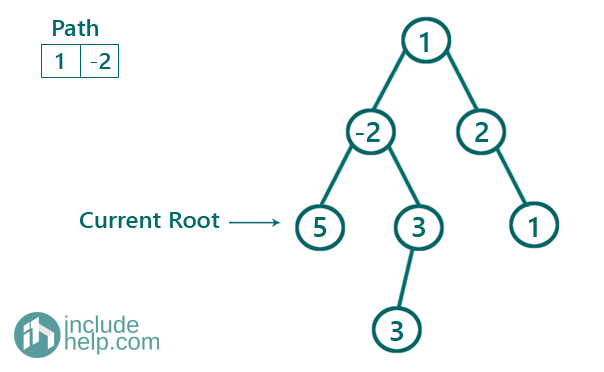Again,

The root is not NULL, so we add root to the vector paths and recur for the left subtree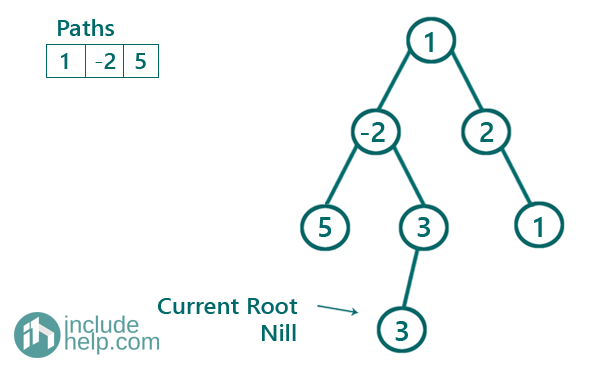Now root is Null, so it returns to the previous calling function. Now, it goes for the right subtree, the right subtree is again NULL

That's why the control again returns to this program and now we find the sum K in the currently stored path vector which is [1, -2, 5]. Here only one path we can find which is the entire path 1->-2->5 which sums up to 4

After printing this step it backtracks and pops 5 out of the path list and then recurs for the right subtree of the root -2.

You can do rest of the dry run by yourself as showed up to now.